## Lenses

Ancient Egyptians, Babylonians, and Greeks, all had a sense of basic optics. That means we have been playing with lenses for a long time. A more specialized form of lenses, spectacles appeared around the 12th century, but it took until contemporaries of Galileo and later Newton to develop a decent telescope.

We should all like lenses. A lot. They save near-sighted folk from waving to strangers thinking that they're mom and far-sighted folk from a life of audio books. So how do lenses work? And why can they fix both near- and far-sightedness?

Lenses, unlike mirrors, generate images through refraction, and by making lenses of different shapes we can change the type of image that lenses "make."

Lenses are a lot more complicated than mirrors because light passes through them instead of just bouncing off them. That means we have to think about what light rays do when they enter the lens and when they exit the lens. In other words, when light passes through a lens, it gets refracted twice: once when it enters the lens and again when it exits.

Lucky for us, we only have to consider thin lenses, which will make things a lot simpler.

The two primary types of thin lenses are:

• convex-converging and
• concave-diverging lenses.

Convex lenses, like convex mirrors, have surfaces that bulge outward. Concave lenses have surfaces that cave in. Try this to remember which is converging and which diverging: Convex is converging. This leaves diverging for the concave mirror. We can also remember how sweet it is that we have diverged from living in caves. Go humans.

We should mention, though, that when we talk about lenses, we always make one important assumption: the image that we are looking at through a lens is at an infinite distance from the lens along the principal axis (see the diagram below). It's easier to predict what light rays will do if they are all parallel when they hit the lens. That only happens when an object is a very long distance away from the lens.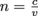Lenses, like mirrors, have a focal point, F. If an object is infinitely far away from the lens or mirror (again, the parallel thing), then the focal point is where the image forms. In the real world, this never happens except, maybe, when we're frying insects with magnifying glasses with an infinitesimally small image (and with the high energy density) of a sun. The lenses in our eyes and eyeglasses do the same thing: take light from a distance of infinity (object distance, that is) and focus it on our (very small) retinas, positioned at the focus of the lens.

Like mirrors, lenses have a focal length, f. The focal length is the distance from the lens to the focal point. Like mirrors, lenses can generate virtual and real images that can be right side up or inverted, true size, magnified, or shrunken.

The similarities between lenses and mirrors don't stop at focal point and focal length. The same comments about parabolic versus spherical surfaces apply, for example. Lenses also have an equation that lets us calculate the focal length of a lens and an equation that lets us calculate the magnification factor of a lens. But worry not, young padawan. No new equations to remember. We use the same equations for lenses that we use for mirrors.

Thin-lens' equation: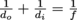.

Magnification equation: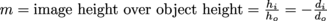Just as with mirrors there are some sign conventions we have to follow. Any object or image that's real is positive:

(1) Object distance, do, and object height, ho, are always positive.
(2) Image distances, di are positive for real images.
(3)Image heights, hi, are positive for real and virtual images that are
right side up.
(4) Image distance, di, negative for virtual images.
(5) Image heights, hi, are negative for real and virtual images that are
upside down.
(6) Focal length, f, is positive for convex (converging) lenses and negative for concave (diverging) lenses.

And lastly, for the magnification equation:

(1) An upside down image has a negative hi and a negative magnification
factor, m.
(2) A right side up image has a positive hi and a positive magnification
factor, m.

Okay, okay, okay. We agree. There's too much to remember. But if we stick with real is positive, we should be able to get the sign conventions right. Now, let's move on to the less memory-heavy parts.

Mirror and lenses both come in either convex flavor or concave flavor, right? Right. Well, with mirror and lenses, we also follow the same basic ray diagram steps.

1. In parallel, out through focus
2. In through focus, out parallel
3. Straight through center (reflected back on itself)

Convex lenses converge light rays similar to (but on the opposite side as) concave mirrors pictures in the diagram below: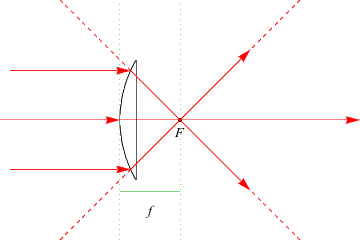Concave lenses diverge light rays similar to convex mirrors, as shown below::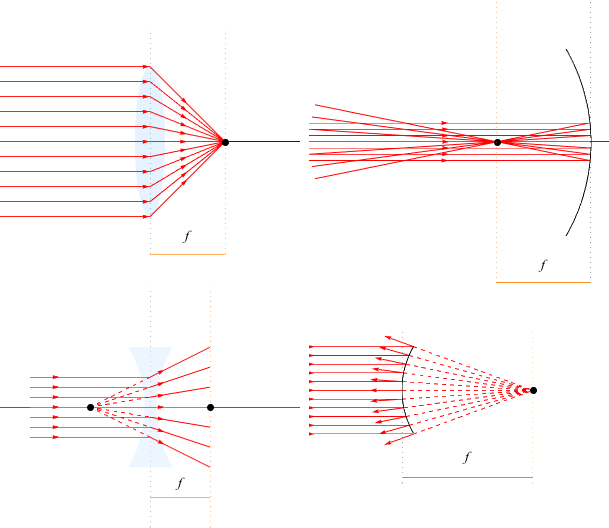Can we tell which of these lenses generates a real image and which virtual images? Remember in the mirror section we learned that virtual images are just that: virtual. Real images are real because they focus light rays on a physical location. Which of these lenses has a focal point that light rays actually pass through and which one a focal point where our brain has filled in the path of the refracted light rays?

The convex lens' focal point is the focal point that the refracted rays actually pass through, so the convex lens generates a real image, as long as the object is beyond the distance of the focal length, the same as for concave mirrors.

The concave lens's focal point is a virtual focal point, a figment of our imagination. Concave mirrors generate virtual images exclusively, just like convex mirrors

We could memorize these facts but why bother when we can always sketch a ray diagram?

Diagrams are our friends.

We can explore why some lenses make images bigger, why the film in a movie projector has to be upside down to get a right side up projected image, and how contact lenses work, all with ray diagrams.

Again, as for concave mirrors, the answer lies in the relative distance between the focal distance and the object. Anyone else sense more ray diagrams coming down the pipeline? The equation gives precise numeric answers, but the diagrams inform us of signs of do and di, and it help us make sense of the system as a whole.

When we draw ray diagrams for lenses we include two focal points because light can pass through the lens from both sides. If the lens is shaped the same on both sides, then the two focal points are same focal length from the lens center.

### Convex Lenses

Let's start by looking at convex lenses. Draw an arrow just past the focal point on the left of the lens.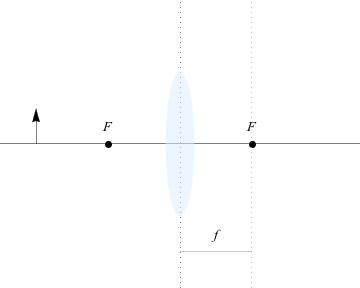Now, draw the first ray running parallel to the principal axis from the top of the arrow, hitting the lens, and bending such that the refracted ray passes through the focal point to the right of the lens.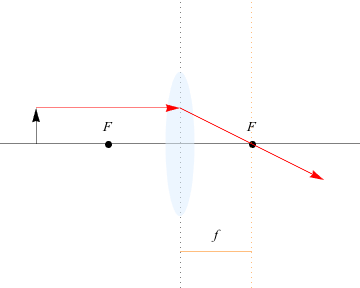Next, we draw a second ray and third ray, one of which runs straight through the center of the lens, also called the optical center, and the other ray run through F on the same side of the arrow and out parallel, intersecting in the same place as the other two rays. The top of the image is marked by the intersection.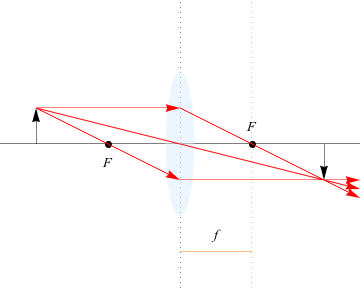We get a real image from light rays converging to form the image. As is typical for all real images, it's upside down. The magnification changes depending on the relative placement between object and focal distance, but this one has a magnification over 1.

Math-break-time!

What's the object distance and magnification for a convex lens with a focal length of 12 inches and an image at 16 inches?

We need to use.Units in inches don't matter for this equation because SI units aren't required to use this one. The image distance is positive, the focal distance is positive, and all that leads to do = 48 inches. That's definitely far beyond the center of the circle, some 24 inches from the lens (from r = 2f). The magnification, now that we've found do, is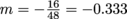The real image produced is a third as tall (and wide) as the object.
Now, back to our regularly scheduled programming.

That was all for an object farther away than the focus. What happens when we place the arrow between the focal point and the lens?

Grab that pencil, or whatever might be left of it after all the drawing we have done, and draw the same three rays we just drew for the last example, with the one for "in through focus" using the line leading from the focus behind the object to the top of the arrow, then out parallel on the opposite side.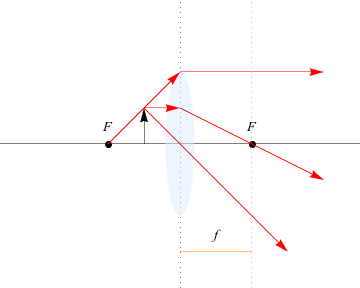Are we scratching our heads yet? We should be. The two rays don't intersect, so how do we determine what image is formed and where?

We do just what we did for mirrors. Our brain extends the two rays to find where they cross and place the image at that point. Sounds like a virtual image coming on, doesn't it? Let's see: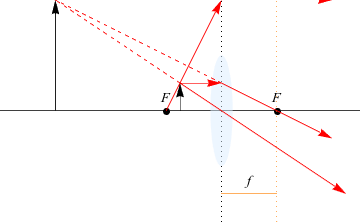Yup, we got a virtual image. Virtual, because the light rays don't pass through it. This image, as all virtual images is right side up, and has a magnification over 1.

Let's work through one more special case. What happens when we place the arrow a distance greater than twice the focal distance away from the lens?

Draw it out. We may be developing the equivalent of tennis elbow from all this drawing (we call it optics wrist) but trust us, all this practice drawing these examples will make drawing ray diagrams second nature in no time. Well, maybe more like fifth. But here we go, same thing as above. Draw the same three rays, our new mantra:

1. In parallel, out through focus
2. In through focus, out parallel
3. Straight through center (reflected back on itself)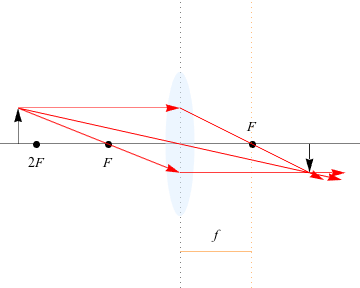This time, the image that is inverted (real) and smaller, with a magnification less than 1. Just as for concave mirrors, there are three types of images that can be formed by a convex lens.

(1) When the object is placed between the focal point and the lens we get an enlarged, right side up, virtual image.
(2) When the object is placed between F and 2F, we get an upside down, enlarged, real object.
(3) When an object is placed at a distance greater than 2F from the lens we get a shrunken, upside down, real image.

Number (2) is how movie projectors work. The film in a movie projector is placed upside down so that the enlarged, real image projected onto the screen is right side up. Why don't we use option (1) to make movie projectors with its right side up, enlarged image? Unfortunately, option (1) generates a virtual image. If we placed a screen at the image point of this set up, you would see nothing because there are no light rays there.

And option (3), how could that be useful? It's what's used in cameras: it's how we can fit the Eiffel Tower on a one-inch piece of film.

### Common Mistakes

Remember that convex lenses are cousins with concave mirrors so the sign conventions and object/image relationships at various distances are the same.

### Brain Snack

Who would have guessed that a convex "lens" was named after lentils? Yes, the delicious (sometimes) legumes. Well, they were.

They do have the same shape, we guess.

### Concave Lenses

We can draw the same kinds of ray diagrams to see what concave lenses do. Just remember, concave lenses diverge light, so instead of focusing ray 1, concave lenses bend light rays outwards as if it came from the focal point on the object side of the lens, but we still use the same three rays as always:

1. In parallel, out through focus
2. In through focus, out parallel
3. Straight through center (reflected back on itself)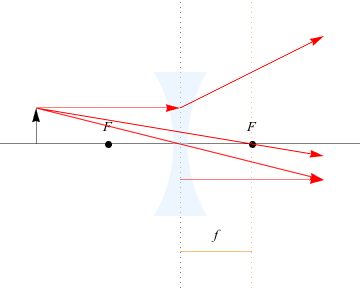As before, when the light rays don't converge, we extend them virtually until they intersect to find the location of the virtual image.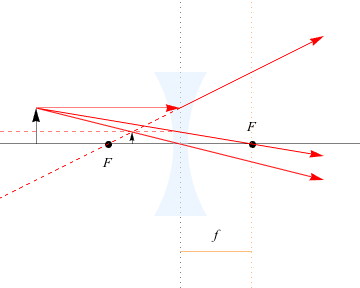We get a virtual image, right side up, but with a magnification less than 1. Next, what happens if we place the half moon between the focal point and the concave lens?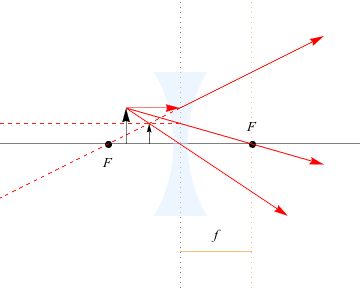Nothing much changes. Simple concave lenses always generate virtual images, right side up, and smaller. They are used to correct various vision problems.

Math Break!

Looks like it's that time again. Where does the image appear for a concave lens with a focal length of 8 cm and an object 5 cm away from it?

Using the equation we use for all curved mirrors and lenses,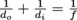,}, we identify that f = -8 (because it's a concave lens) and do = 5. We rearrange the formula into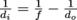to relatively isolate di. Solving, di = -3.1 cm. That's as it should be: virtual images have negative distances. If we were given the image distance as 3.1 with a concave lens, we'd still substitute in di = -3.1 cm.

Now, back to our regularly scheduled physics lesson.

We find concave lenses in peep holes in doors. You see a much smaller version of the person standing in front of your door, and since it's also right side up, it's virtual.

### Common Mistakes

Remember that concave lenses act an awful lot like a convex mirror. We use sign conventions and ray diagram extensions to find the location of a virtual image for both.

### Brain Snack

Concave mirrors are used to correct nearsightedness. Read here to see what that has to do with Lord of the Flies.